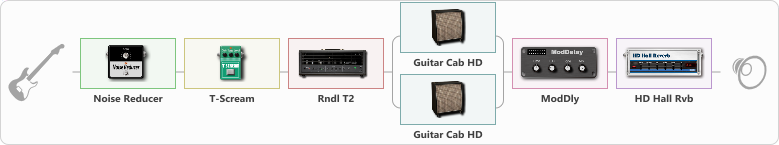# metallica

Discussion in 'ToneLib-GFX presets' started by Alexandr Shtorm, Mar 20, 2023.

1. metallica

Preset name: Wild Beast

Effects chain:Effect: "Noise Reducer" (Dynamics / Filter), active - "yes"
{
"Sens" = 80
"Mode" = Hard
}

Effect: "T-Scream" (Overdrive / Distortion), active - "yes"
{
"Drive" = 15
"Tone" = 80
"Level" = 73
}

Effect: "Rndl T2" (Amp simulators), active - "yes"
{
"Gain" = 80
"Bass" = 45
"Middle" = 45
"Treble" = 60
"Presence" = 45
"Master" = 65
"Level (dB)" = 0
}

Effect: "Splitter" (Dynamics / Filter)
{
"A-Bypass" = Off
"A-Pan" = 26
"A-Level" = 55
"B-Bypass" = Off
"B-Pan" = -24
"B-Level" = 55
"Width" = 56

'A' branch:
{

Effect: "Guitar Cab HD" (Cabinets), active - "yes"
{
"Model" = Peavey 5150 (4x12")
"Mic Model" = Dynamic SM57
"Mic Position" = Middle
"Mic Distance" = Near
"Low Cut (Hz)" = 80
"Hi Cut (kHz)" = 20.0
"Mix" = 100
"Level (dB)" = 0
}
}
'B' branch:
{

Effect: "Guitar Cab HD" (Cabinets), active - "yes"
{
"Model" = Orange PPC (4x12")
"Mic Model" = Dynamic MD421
"Mic Position" = Middle
"Mic Distance" = Middle
"Low Cut (Hz)" = 80
"Hi Cut (kHz)" = 20.0
"Mix" = 100
"Level (dB)" = 0
}
}
}

Effect: "ModDly" (Delay), active - "yes"
{
"Time" = 457
"Feedback" = 39
"Tone" = 70
"Speed" = 1.6
"Mix" = 40
}

Effect: "HD Hall Rvb" (Reverberation), active - "yes"
{
"Time" = 5.8
"PreLPF" = 44
"PreDelay" = 96
"HiDamp" = 27
"LoGain" = -3.3
"Mix" = 9
}

Note: You will need to download and install the ToneLib-GFX software to use the preset.

#### Attached Files:

• ###### metallica.tlgfx
File size:
815 bytes
Views:
1,496
SOLNYAK likes this.